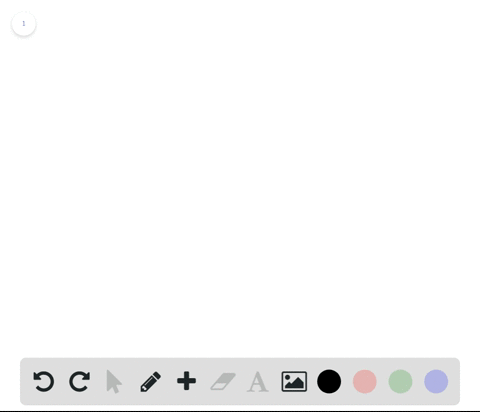Enroll in one of our FREE online STEM bootcamps. Join today and start acing your classes!View Bootcamps00:14
Problem 76

# True or False? In Exercises $73-76$ , determine whether the statement is true or false. If it is false, explain why or give an example that shows it is false.If $$\lim _{x \rightarrow c} f(x)=L, then f(c)=L$$

## Discussion

You must be signed in to discuss.

## Video Transcript

in this problem here, ASA. True or false questions? Four minutes X approaches si for the function F of X is equal to l. Then f c is equal to l. So basically, this question is asking if the limit exists. Is it equal to the function value? At that point in our claim is that this statement is false. Show this is false will provide a counter example to the claim. So let's look at the graph here and let's look at so get X equals two. It's kind of look at this area here. So since we're considering thanks equals two, then we can see that. Sure, the limit as X approaches to seems to be this. Why by you right here from the left and from the right. So that limits approximately 0.5. However, let's see what isthe I have to. Is it equal to the limit? Well, if you look at the graph, we have this filled in circle here, which means the function does not take on that. Why cordon at that point? And there's no film, not anywhere else. So we can actually see that f of two is not to find so f of two is certainly not equal to Is there a 0.5, which is the function value start, which is the limit. So it is not in the case that wanna limit exists, that it has to be equal to the value of the function at that port? Yeah.